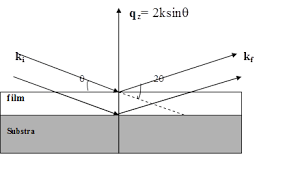# How to Calculate and Solve for Resolution X-rays | X-Ray CrystallographyThe image above represents resolution x-rays.

To compute for resolution x-rays, two essential parameters are needed and these parameters are Average Height of Voltage Pulse (V) and Spread of Voltage Pulse (δv).

The formula for calculating resolution x-rays:

R% = 100 (√(δv)/V)

Where:

R% = Resolution X-rays
V = Average Height of Voltage Pulse
δv = Spread of Voltage Pulse

Let’s solve an example;
Find the resolution x-rays when the average height of voltage pulse is 21 and the spread of voltage pulse is 10.

This implies that;

V = Average Height of Voltage Pulse = 21
δv = Spread of Voltage Pulse = 10

R% = 100 (√(δv)/V)
R% = 100 (√(10)/21)
R% = 100 (3.162/21)
R% = 100 (0.15)
R% = 15.05

Therefore, the resolution x-rays is 15.05%.

Calculating the Average Height of Voltage Pulse when the Resolution X-rays and the Spread of Voltage Pulse is Given.

V = 100 x √(δv) / R%

Where;

V = Average Height of Voltage Pulse
R% = Resolution X-rays
δv = Spread of Voltage Pulse

Let’s solve an example;
Find the average height of voltage pulse when the resolution x-rays is 40 and the spread of voltage pulse is 4.

This implies that;

R% = Resolution X-rays = 40
δv = Spread of Voltage Pulse = 4

V = 100 x √(δv) / R%
V = 100 x √(4) / 40
V = 100 x 2 / 40
V = 200 / 40
V = 5

Therefore, the average height of voltage pulse is 5.

Calculating the Spread of Voltage Pulse when the Resolution X-rays and the Average Height of Voltage Pulse is Given.

δv = √(R%V / 100)

Where;

δv = Spread of Voltage Pulse
R% = Resolution X-rays
V = Average Height of Voltage Pulse

Let’s solve an example;
Find the spread of voltage pulse when the resolution x-rays is 42 and the average height of voltage pulse is 16.

This implies that;

R% = Resolution X-rays = 42
V = Average Height of Voltage Pulse = 16

δv = √(R%V / 100)
δv = √(42 x 16 / 100)
δv = √(672 / 100)
δv = √(6.72)
δv = 2.59

Therefore, the spread of voltage pulse is 2.59.

Nickzom Calculator – The Calculator Encyclopedia is capable of calculating the resolution x-rays.

To get the answer and workings of the resolution x-rays using the Nickzom Calculator – The Calculator Encyclopedia. First, you need to obtain the app.

You can get this app via any of these means:

To get access to the professional version via web, you need to register and subscribe for NGN 2,000 per annum to have utter access to all functionalities.
You can also try the demo version via https://www.nickzom.org/calculator

Apple (Paid) – https://itunes.apple.com/us/app/nickzom-calculator/id1331162702?mt=8
Once, you have obtained the calculator encyclopedia app, proceed to the Calculator Map, then click on Materials and Metallurgical under Engineering.Now, Click on X-ray Crystallography under Materials and MetallurgicalNow, Click on Resolution X-rays under X-ray CrystallographyThe screenshot below displays the page or activity to enter your values, to get the answer for the resolution x-rays according to the respective parameters which is the Average Height of Voltage Pulse (V) and Spread of Voltage Pulse (δv).Now, enter the value appropriately and accordingly for the parameter as required by the Average Height of Voltage Pulse (V) is 21 and Spread of Voltage Pulse (δv) is 10.Finally, Click on CalculateAs you can see from the screenshot above, Nickzom Calculator– The Calculator Encyclopedia solves for the resolution x-rays and presents the formula, workings and steps too.